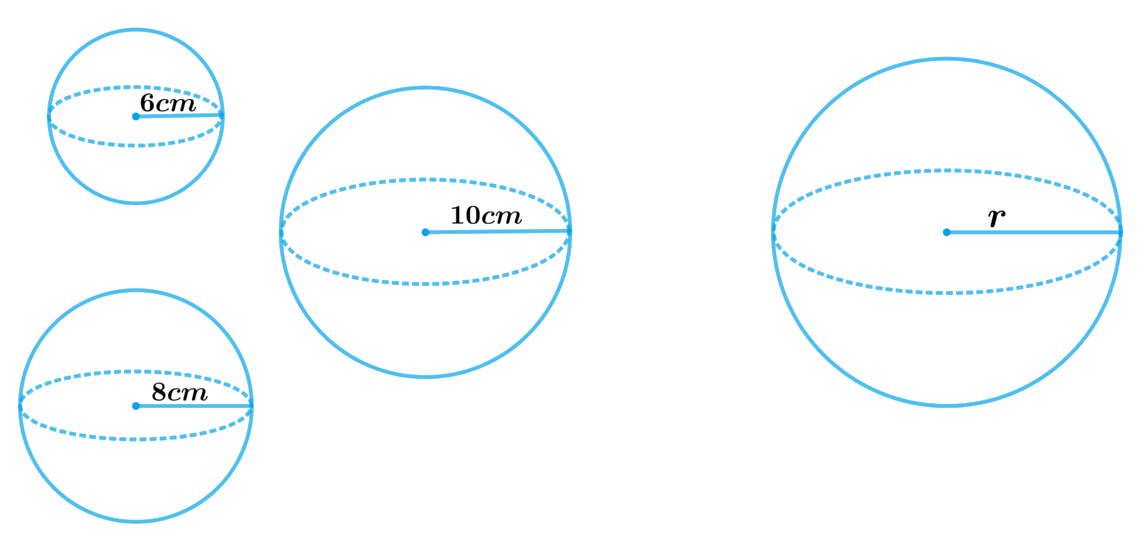# Ex.13.3 Q2 Surface Areas and Volumes Solution - NCERT Maths Class 10

Go back to  'Ex.13.3'

## Question

Metallic spheres of radii $$6 \,\rm{cm}$$, $$8\, \rm{cm}$$, and $$10\, \rm{cm}$$, respectively, are melted to form a single solid sphere. Find the radius of the resulting sphere.

## Text Solution

What is known?

Radii of three  metallic spheres are $$6\, \rm{cm}$$, $$8\, \rm{cm}$$ and $$10 \,\rm{cm}$$

What is unknown?

The radius of the resulting sphere

Reasoning:

Draw a figure to visualize the shapes betterSince, three metallic spheres are melted and recast into a single solid sphere then the sphere formed by recasting these spheres will be same in volume as the sum of the volumes of these spheres.

Volume of the resulting sphere $$=$$ Sum of the volumes of three spheres

We will find the volume of the sphere by using formula;

Volume of the sphere\begin{align} = \frac{4}{3}\pi {r^3}\end{align}

where $$r$$ is the radius of the sphere

Steps:

Radius of 1st sphere,$${r_1} = 6cm$$

Radius of 2nd sphere,$${r_2} = 8cm$$

Radius of 3rd sphere,$${r_3} = 10cm$$

Let the radius of the resulting sphere be $$r$$.

Volume of the resulting sphere $$=$$ Sum of the volumes of three spheres

\begin{align}\frac{4}{3}\pi {r^3} &= \frac{4}{3}\pi r_1^3 + \frac{4}{3}\pi r_2^3 + \frac{4}{3}\pi r_3^3\\ \frac{4}{3}\pi {r^3} &= \frac{4}{3}\pi \left[ {r_1^3 + r_2^3 + r_3^3} \right]\\{r^3} &= \left[ {r_1^3 + r_2^3 + r_3^3} \right]\\{r^3} &= \left[ {{{\left( {6cm} \right)}^3} + {{\left( {8cm} \right)}^3} + {{\left( {10cm} \right)}^3}} \right]\\{r^3} &= \left[ {216c{m^3} + 512c{m^3} + 1000c{m^3}} \right]\\{r^3} &= 1728c{m^3}\\r &= 12cm\end{align}

Therefore, the radius of the sphere so formed will be $$12 \rm{cm.}$$

Learn from the best math teachers and top your exams

• Live one on one classroom and doubt clearing
• Practice worksheets in and after class for conceptual clarity
• Personalized curriculum to keep up with school